Courses

# Circles - NCERT Solution, Class 10 Mathematics Class 10 Notes | EduRev

## Class 10 : Circles - NCERT Solution, Class 10 Mathematics Class 10 Notes | EduRev

The document Circles - NCERT Solution, Class 10 Mathematics Class 10 Notes | EduRev is a part of Class 10 category.
All you need of Class 10 at this link: Class 10

## Circle

Exercise 1

Question: 1. How many tangents can a circle have?

Solution: On a given point of the perimeter there can be one and only one tangent. Otherwise there can be infinitely many tangents.

Question: 2. Fill in the blanks :

(i) A tangent to a circle intersects it in point (s).

Solution:- One

(ii) A line intersecting a circle in two points is called a.

Solution:- Secant

(iii) A circle can have parallel tangents at the most.

Solution: Two

(iv) The common point of a tangent to a circle and the circle is called .

Solution: Point of contact

Question: 3. A tangent PQ at a point P of a circle of radius 5 cm meets a line through the centre O at a point Q so that OQ = 12 cm. Length PQ is :

(A) 12 cm (B) 13 cm (C) 8.5 cm (D)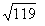cm.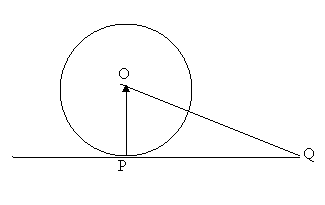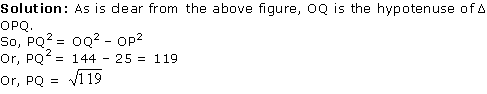Exercise 2

Question: 1. From a point Q, the length of the tangent to a circle is 24 cm and the distance of Q from the centre is 25 cm. The radius of the circle is

(A) 7 cm (B) 12 cm (C) 15 cm (D) 24.5 cmSolution: In Δ OPQ

OP2 = OQ2 – PQ2

Or, OP2 = 252 – 242

Or, OP2 = 625 – 576

Or, OP2 = 49

Or, OP = 7

2. In the following figure, if TP and TQ are the two tangents to a circle with centre O so that angle POQ = 110°, then angle PTQ is equal to

(A) 60° (B) 70° (C) 80° (D) 90°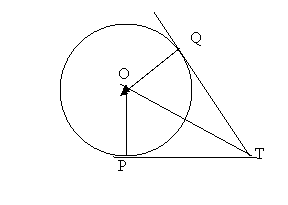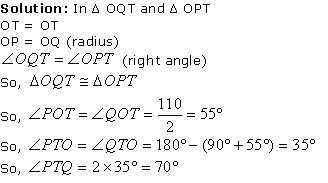3. If tangents TQ and TP from a point T to a circle with centre O are inclined to each other at angle of 80°, then angle TOP is equal to

(A) 50° (B) 60° (C) 70° (D) 80°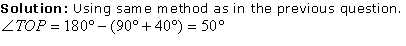4. Prove that the tangents drawn at the ends of a diameter of a circle are parallel.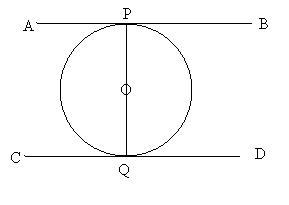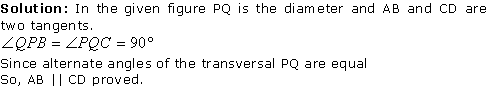5. Prove that the perpendicular at the point of contact to the tangent to a circle passes through the centre.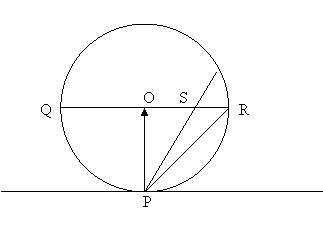Solution: In the given figure, QR is diameter and O is centre. OP, SP and RP are lines touching the tangent at the point of contact.

In triangles POS and POR

Line PS > PO and SO

Line PR > PO and RO

So, PS and PR are hypotenuse of respective triangles.

So, it is proved that Angle POR = Right Angle

If PO is increased to reach the other end of the perimeter, then it will be the diameter.

6. The length of a tangent from a point Q at distance 5 cm from the centre of the circle is 4 cm. Find the radius of the circle.Solution: In Δ OPQ

OP2 = OQ2 – PQ2

Or, OP2 = 25 – 16 = 9

Or, OP = 3

7. Two concentric circles are of radii 5 cm and 3 cm. Find the length of the chord of the larger circle which touches the smaller circle.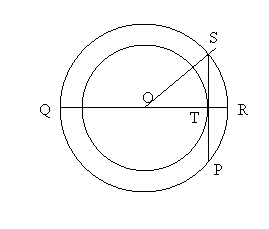Solution: OS = 5 cm

OT = 3 cm

ST2 = OS2 – OT2

Or, ST2 = 25 – 9 = 16

Or, ST = 4

So, SP = 8 cm

8. A quadrilateral ABCD is drawn to circumscribe a circle. Prove that

AB + CD = AD + BC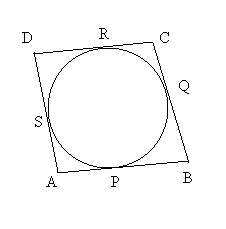Solution: AP = AS (tangents from a point are always equal)

Similarly,

PB = BQ

CQ = CR

DR = DS

AB = AP + PB

BC = BQ + CQ = PB + CQ

CD = CR + DR = CQ + DR

AD = DS + AS = DR + AP

AB + CD = AP + PB + CQ + DR ………….. (i)

AD + BC = DR + AP + PB + CQ …………… (ii)

From equation (i) and (ii) it is clear

AB + CD = AD + BC

9. In the given figure, XY and X′Y′ are two parallel tangents to a circle with centre O and another tangent AB with point of contact C intersecting XY at A and X′Y′ at B. Prove that angle AOB = 90°.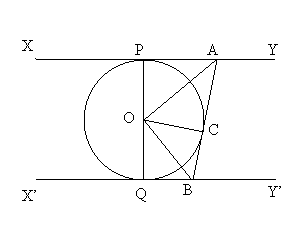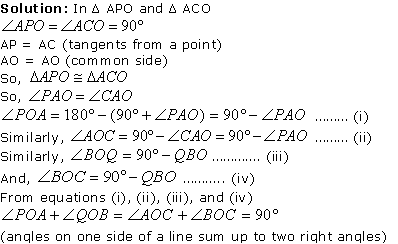10. Prove that the angle between the two tangents drawn from an external point to a circle is supplementary to the angle subtended by the line-segment joining the points of contact at the centre.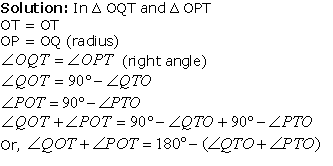So, it is clear that angle subtended by TP and TQ is supplementary to angle subtended by OP and OQ.

11. Prove that the parallelogram circumscribing a circle is a rhombus.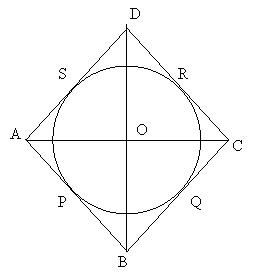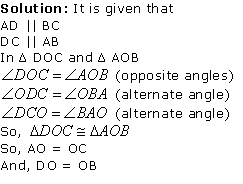As diagonals are bisecting each other, so the given parallelogram is a rhombus.

Offer running on EduRev: Apply code STAYHOME200 to get INR 200 off on our premium plan EduRev Infinity!

,

,

,

,

,

,

,

,

,

,

,

,

,

,

,

,

,

,

,

,

,

,

,

,

;